# UNIT 8 lesson 8.1 grade 7.pptx

May. 27, 2023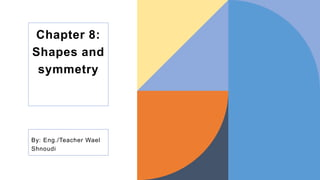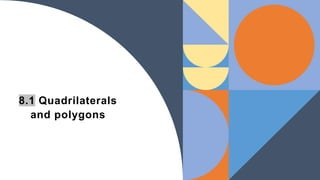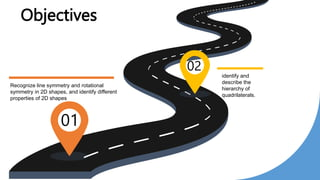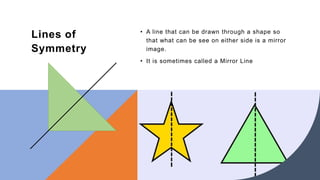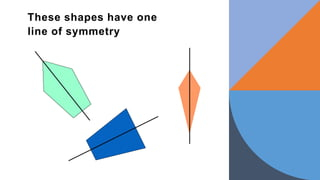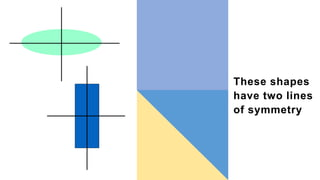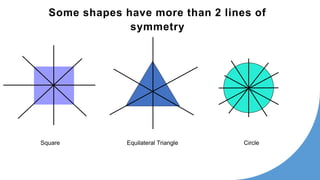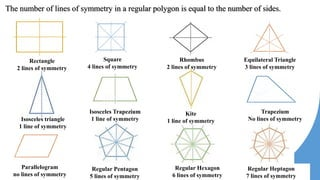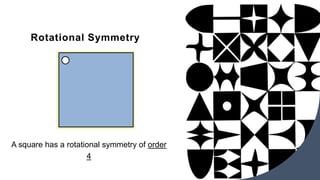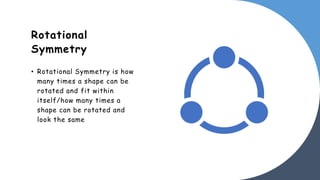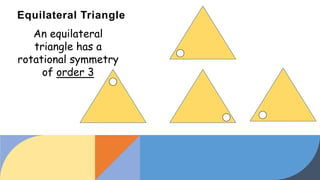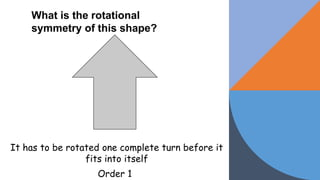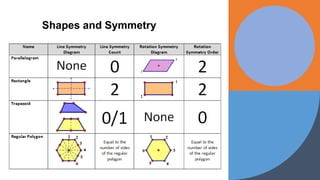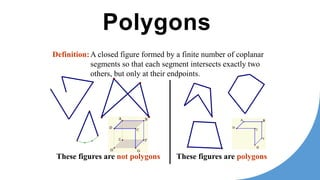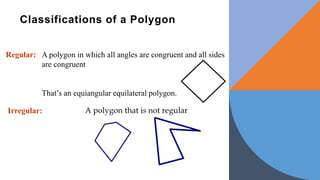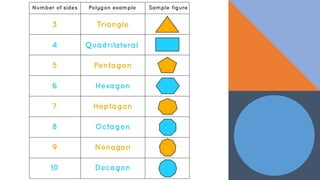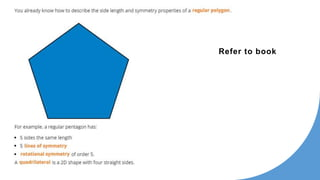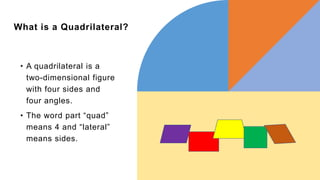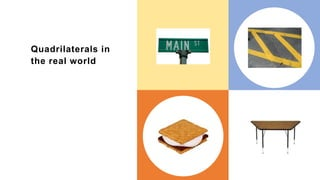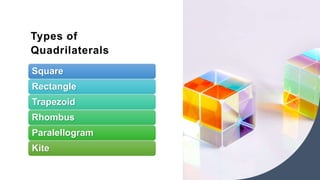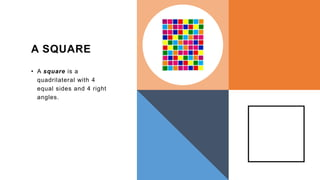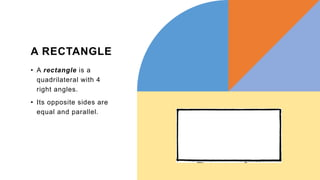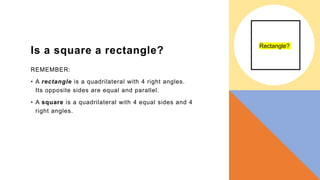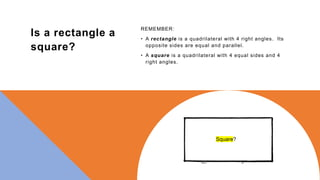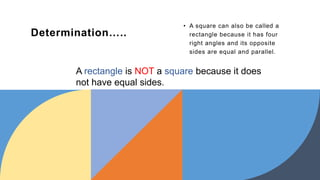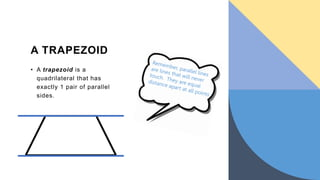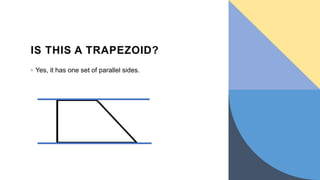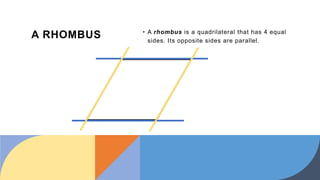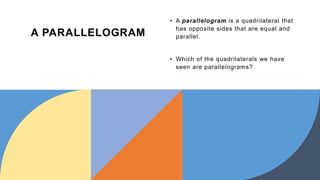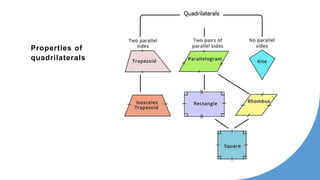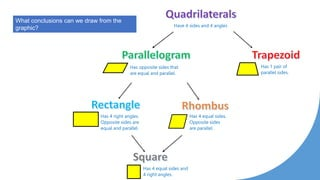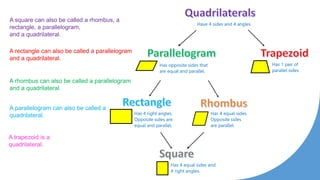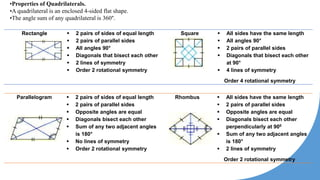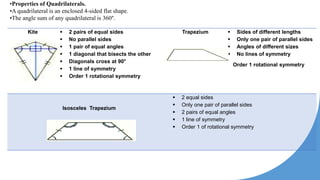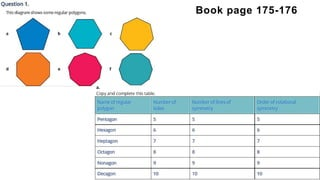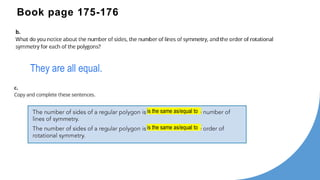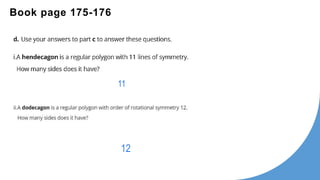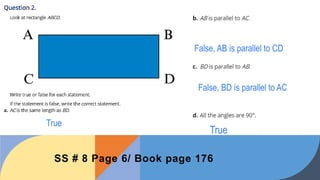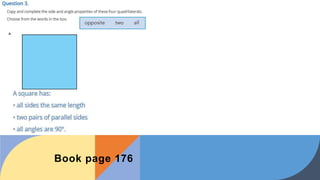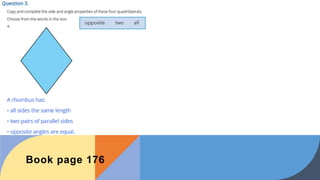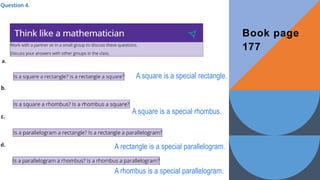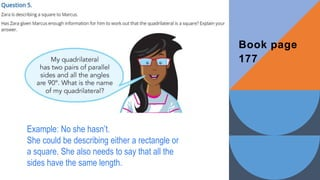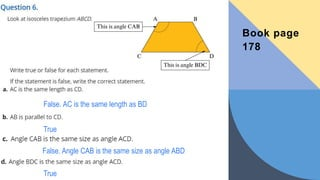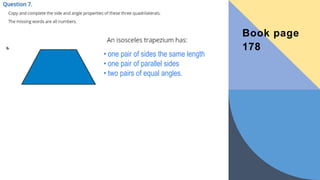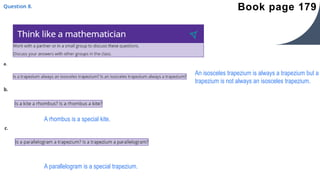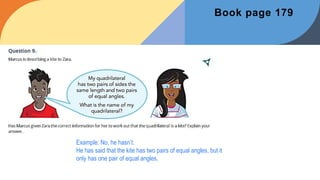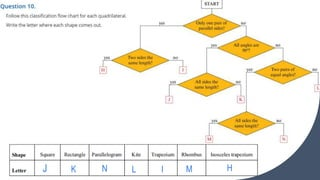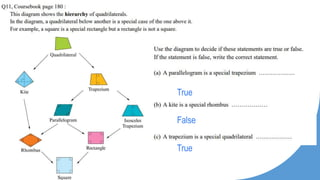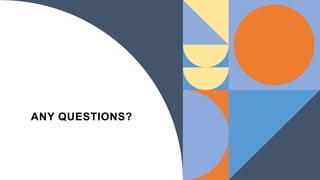1 of 49

### UNIT 8 lesson 8.1 grade 7.pptx

• 1. Chapter 8: Shapes and symmetry By: Eng./Teacher Wael Shnoudi
• 2. 8.1 Quadrilaterals and polygons
• 3. Objectives 01 02 Recognize line symmetry and rotational symmetry in 2D shapes, and identify different properties of 2D shapes identify and describe the hierarchy of quadrilaterals.
• 4. Lines of Symmetry • A line that can be drawn through a shape so that what can be see on either side is a mirror image. • It is sometimes called a Mirror Line
• 5. These shapes have one line of symmetry
• 6. These shapes have two lines of symmetry
• 7. Some shapes have more than 2 lines of symmetry Square Equilateral Triangle Circle
• 8. Rectangle 2 lines of symmetry Square 4 lines of symmetry Rhombus 2 lines of symmetry Equilateral Triangle 3 lines of symmetry Isosceles triangle 1 line of symmetry Isosceles Trapezium 1 line of symmetry Kite 1 line of symmetry Trapezium No lines of symmetry Parallelogram no lines of symmetry Regular Pentagon 5 lines of symmetry Regular Hexagon 6 lines of symmetry Regular Heptagon 7 lines of symmetry The number of lines of symmetry in a regular polygon is equal to the number of sides.
• 9. Rotational Symmetry A square has a rotational symmetry of order 4
• 10. Rotational Symmetry • Rotational Symmetry is how many times a shape can be rotated and fit within itself/how many times a shape can be rotated and look the same
• 11. Equilateral Triangle An equilateral triangle has a rotational symmetry of order 3
• 12. What is the rotational symmetry of this shape? It has to be rotated one complete turn before it fits into itself Order 1
• 13. Shapes and Symmetry
• 14. These figures are not polygons These figures are polygons Definition:A closed figure formed by a finite number of coplanar segments so that each segment intersects exactly two others, but only at their endpoints. Polygons
• 15. Classifications of a Polygon Regular: A polygon in which all angles are congruent and all sides are congruent That’s an equiangular equilateral polygon. Irregular: A polygon that is not regular
• 17. Refer to book
• 18. What is a Quadrilateral? • A quadrilateral is a two-dimensional figure with four sides and four angles. • The word part “quad” means 4 and “lateral” means sides.
• 19. Quadrilaterals in the real world
• 20. Types of Quadrilaterals Square Rectangle Trapezoid Rhombus Paralellogram Kite
• 21. A SQUARE • A square is a quadrilateral with 4 equal sides and 4 right angles.
• 22. A RECTANGLE • A rectangle is a quadrilateral with 4 right angles. • Its opposite sides are equal and parallel.
• 23. Is a square a rectangle? REMEMBER: • A rectangle is a quadrilateral with 4 right angles. Its opposite sides are equal and parallel. • A square is a quadrilateral with 4 equal sides and 4 right angles. Rectangle?
• 24. Is a rectangle a square? REMEMBER: • A rectangle is a quadrilateral with 4 right angles. Its opposite sides are equal and parallel. • A square is a quadrilateral with 4 equal sides and 4 right angles. Square?
• 25. Determination….. • A square can also be called a rectangle because it has four right angles and its opposite sides are equal and parallel. A rectangle is NOT a square because it does not have equal sides.
• 26. A TRAPEZOID • A trapezoid is a quadrilateral that has exactly 1 pair of parallel sides.
• 27. IS THIS A TRAPEZOID? ◦ Yes, it has one set of parallel sides.
• 28. A RHOMBUS • A rhombus is a quadrilateral that has 4 equal sides. Its opposite sides are parallel.
• 29. A PARALLELOGRAM • A parallelogram is a quadrilateral that has opposite sides that are equal and parallel. • Which of the quadrilaterals we have seen are parallelograms?
• 31. Have 4 sides and 4 angles Has opposite sides that are equal and parallel. Has 1 pair of parallel sides. Has 4 right angles. Opposite sides are equal and parallel. Has 4 equal sides. Opposite sides are parallel. Has 4 equal sides and 4 right angles. What conclusions can we draw from the graphic?
• 32. A square can also be called a rhombus, a rectangle, a parallelogram, and a quadrilateral. A rectangle can also be called a parallelogram and a quadrilateral. A rhombus can also be called a parallelogram and a quadrilateral. A parallelogram can also be called a quadrilateral. A trapezoid is a quadrilateral. Have 4 sides and 4 angles Has opposite sides that are equal and parallel. Has 1 pair of parallel sides. Has 4 right angles. Opposite sides are equal and parallel. Has 4 equal sides. Opposite sides are parallel. Has 4 equal sides and 4 right angles.
• 33. Rectangle  2 pairs of sides of equal length  2 pairs of parallel sides  All angles 90°  Diagonals that bisect each other  2 lines of symmetry  Order 2 rotational symmetry Square  All sides have the same length  All angles 90°  2 pairs of parallel sides  Diagonals that bisect each other at 90°  4 lines of symmetry Order 4 rotational symmetry •Properties of Quadrilaterals. •A quadrilateral is an enclosed 4-sided flat shape. •The angle sum of any quadrilateral is 360°. Parallelogram  2 pairs of sides of equal length  2 pairs of parallel sides  Opposite angles are equal  Diagonals bisect each other  Sum of any two adjacent angles is 180°  No lines of symmetry  Order 2 rotational symmetry Rhombus  All sides have the same length  2 pairs of parallel sides  Opposite angles are equal  Diagonals bisect each other perpendicularly at 900  Sum of any two adjacent angles is 180°  2 lines of symmetry Order 2 rotational symmetry
• 34. •Properties of Quadrilaterals. •A quadrilateral is an enclosed 4-sided flat shape. •The angle sum of any quadrilateral is 360°. Kite  2 pairs of equal sides  No parallel sides  1 pair of equal angles  1 diagonal that bisects the other  Diagonals cross at 90°  1 line of symmetry  Order 1 rotational symmetry Trapezium  Sides of different lengths  Only one pair of parallel sides  Angles of different sizes  No lines of symmetry Order 1 rotational symmetry Isosceles Trapezium  2 equal sides  Only one pair of parallel sides  2 pairs of equal angles  1 line of symmetry  Order 1 of rotational symmetry
• 35. Book page 175-176
• 36. They are all equal. is the same as/equal to is the same as/equal to Book page 175-176
• 37. 11 12 Book page 175-176
• 38. True False, AB is parallel to CD False, BD is parallel to AC True SS # 8 Page 6/ Book page 176
• 39. Book page 176
• 40. Book page 176
• 41. A square is a special rectangle. A rectangle is a special parallelogram. A rhombus is a special parallelogram. A square is a special rhombus. Book page 177
• 42. Example: No she hasn’t. She could be describing either a rectangle or a square. She also needs to say that all the sides have the same length. Book page 177
• 43. False. AC is the same length as BD True False. Angle CAB is the same size as angle ABD True Book page 178
• 44. • one pair of sides the same length • one pair of parallel sides • two pairs of equal angles. Book page 178
• 45. An isosceles trapezium is always a trapezium but a trapezium is not always an isosceles trapezium. A rhombus is a special kite. A parallelogram is a special trapezium. Book page 179
• 46. Example: No, he hasn’t. He has said that the kite has two pairs of equal angles, but it only has one pair of equal angles. Book page 179
• 47. J K N L I M H
• 48. True False True
• 49. ANY QUESTIONS?